# KSEEB Solutions for Class 9 Science Chapter 10 Gravitation

## Karnataka Board Class 9 Science Chapter 10 Gravitation

### KSEEB Solutions Class 9 Science Chapter 10 Intext Questions

Question 1.
State the universal law of gravitation.
Every object in the universe attracts every other object with a force which is proportional to the product of their masses and inversely proportional to the square of the distance between them. The force is along the line joining the centres of two objects.Question 2.
Write the formula to find the magnitude of the gravitational force between the earth and an object on the surface of the earth.Question 3.
What do you mean by free fall?
Whenever objects fall towards the earth under the gravitational force alone, we say that the objects are in free fall.

Question 4.
What do you mean by the acceleration due to gravity?
Whenever an object falls towards the earth, an acceleration is involved. This acceleration is due to the earth’s gravitational force. Therefore this acceleration is called the acceleration due to the gravitational force of the earth.

Question 5.
What are the differences between the mass of an object and its weight?

 Mass Weight Mass of an object is the measure of the inertia. The weight of an object is the force with which it is attracted to the earth.

Question 6.
Why is the weight of an object on the moon l/6th its weight on the earth?
Mass of moon is lesser than that of earth. Due to this the moon exerts lesser force of attraction on objects. Hence weight of an object on the moon l/6th its weight on the earth.

Question 7.
Why is it difficult to hold a school bag having a strap made of a thin and strong string?
It is difficult to hold a school bag having a starp made of a thin and strong string because the area and starp is small. Hence large pressure is exerted by the starp on the fingers.

Question 8.
What do you mean by buoyancy?
The upward force exerted by the water on the bottle is known as upthrust or buoyant force.

Question 9.
Why does an object float or sink when placed on the surface of the water?
Upward thrust of liquids, (different-in liquid densities) Sink of an object depends upon density. The density of an object is more than water then it sinks. Object density is less than that of water it floats.

Question 10.
You find your mass to be 42 kg on a weighing machine. Is your mass more or less than 42 kg?
Our mass is more than 42 kg.

Question 11.
You have a bag of cotton and an iron bar, each indicating a mass of 100 kg when measured on a weighing machine. In reality, one is heavier than the others. Can you say which one is heavier and why?
Iron bar is heavier than the cotton bag because for the mass the iron bar will have a lesser surface area and apply more force, so the iron bar is heavier than a cotton bag.

### KSEEB Solutions for Class 9 Science Chapter 10 Textbook Exercises

Question 1.
How does the force of gravitation between two objects change when the distance between them is reduced to half?
Force of gravitation between two objects.i.e. the force of gravitation becomes 4 times than the original value.

Question 2.
Gravitational force acts on all objects in proportion, to their masses. Why then a heavy object does not fall faster than a light object?
Each object falls towards the earth with the acceleration equal to the acceleration due to gravity, which is constant (9.8ms-2) and does not depend on the mass of the object. So heavy object does not fall faster than the light object.

Question 3.
What is the magnitude of the gravitational force between the earth and a 1 kg object on its surface? (Mass of the earth is 6 x 1023 kg and radius of the earth
6.4 x 106m)
Gravitational force between the earth and an F = $$\frac{\mathrm{GM}_{\mathrm{m}}}{\mathrm{R}^{2}}$$
Where G = Gravitational constant = 6.67 × 10-11 Nm2kg-2.
M = Mass of earth = 6 × 1024 kg.
R = Radius of earth = 6.4 × 106m
m = Mass of object = 1 kg.
Hence F = $$\frac{6.67 \times 10^{-11} \times 6 \times 10^{24} \times 1}{\left(6.4 \times 10^{6}\right)^{2}}$$
= 9.8N.

Question 4.
The earth and the moon are attracted to each other by gravitational force. Does the earth attract the moon with a force that is greater or smaller or the same as the force with which the moon attracts the earth? Why?
When two objects attract each other then the gravitational force of attraction applied by first object on the second object is same as the force applied by the second object on the first object. So both earth and moon attract each other by the same gravitational force of attraction.

Question 5.
If the moon attracts the earth, why does the earth not move towards the moon?
The earth does not move towards the moon inspite of the attraction by moon because the force with which moon attracts the earth is same by which earth attracts the moon. So net force on the system becomes zero.

Question 6.
What happens to the force between two objects, if
i) the mass of one object is doubled?
ii) the distance between the objects is doubled and tripled?
iii) The masses of both objects are doubled?
Force of attraction between two objects.
F = $$\frac{\mathrm{G} \mathrm{m}_{1} \mathrm{m}_{2}}{\mathrm{r}^{2}}$$
where m1, m2 = masses of the objects
r = Distance between the objects.
G = Gravitational constant.

(i) If mass of one object is doubled, then the new force∴ force becomes double.

(ii) If the distance between the objects is tripled, then new force∴ force becomes one-ninth.

iii) If masses of both objects are doubled, then new force .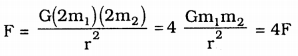i.e. force becomes four times.

Question 7.
What is the importance of universal law of gravitation?
The importance of universal law of gravitation can be understood from the following points:

• All the planets revolve around the sun due to gravitational force between the sun and planets. Hence gravitational force is responsible for the existence of solar system.
• Tides in oceans are formed due to the gravitational force between the moon and water in oceans.
• The atmosphere of the earth is attached with it due to gravitational force of earth.
• Artificial satellites revolve around the earth due to gravitational force of earth.
• We can stand on the surface of earth due to gravitational force of earth.

Question 8.
What is the acceleration of free fall?
The acceleration produced in the motion of an object when it falls freely towards the earth is termed acceleration of free fall. It is also called acceleration due to gravity. Its value on the earth’s surface is 9.8 ms-2.

Question 9.
What do we call the gravitational force between the earth and an object?
The gravitational force between the earth and an object is called the force of gravity.

Question 10.
Amit buys few grams of gold at the poles as per the instruction of one of his friends. He hands over the same when he meets him at the equator will the friend agree with the weight of Gold bought? If not, why? (Hint: The value of g is greater at the poles than at the equator)
Weight of an object, W = mg.
where g = acceleration due to gravity.
The value of g is greater at the poles than at the equator. So the weight of the gold at the equator will be less than the weight of the gold at poles. So Amit’s friend will find the weight of the gold less than the weight told by Amit.

Question 11.
Why will a sheet of paper fall slower than one that is crumpled into a ball?
The sheet of paper falls slower than that is compelled into a ball because in the first case the area of the sheet is more. So it experiences a large opposing force due to air. while the sheet crumpled into a ball experience less opposing force due to small area.

Question 12.
The gravitational force on the surface of the moon is only 1/6 as strong as gravitational force on the earth. What is the weight in newtons of a 10 kg object on the moon and on the earth?
Mass of the object m = 10 kg.
Weight on the earth W = mg.
= 10 × 9.8 = 98 N
Weight on the moon = $$\frac{1}{6}$$ of the weight on the earth.
= 1 × 98 = 16.33 N
= 16.33 N.

Question 13.
A ball is thrown vertically upwards with a velocity of 49m/s. Calculate.
i) the maximum height to which it rises.
ii) the total time it takes to return to the surface of the earth.
Initial velocity u = 49 ms-1
(i) At the maximum height, velocity becomes zero.
∴ Final velocity v = 0
From equation of upward motion
v2 = u2 – 2gh
0 = (49)2 – 2 × 9.8 × h
∴ h = $$\frac{(49)^{2}}{2 \times 9.8}$$ = 1225 m
Maximum height attained = 122.5 m.

(ii) Time is taken by the ball to reach the maximum height.
From equation of upward motion
v = u – gt
0 = 49 – 9.8 × t
∴ t = $$\frac{49}{9.8}$$ = 5s
In the motion against gravity, the time of descent is same as the time of ascent.
So time taken by the ball to fall from maximum height = 5s.
∴ Total time taken by the ball to return to surface of the earth = 5 + 5 = 10s.

Question 14.
A stone is released from the top of a tower of height 19.6 m. Calculate its final velocity just before touching the ground.
h = 19.6 m.
Initial velocity u = 0 (∵ It starts from rest)
From equation of motion.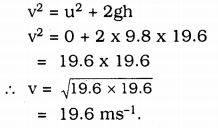Final velocity of stone before touching the ground = 19.6 ms-1.

Question 15.
A stone is thrown vertically upward with an initial velocity of 40m/s. Taking g = 10m/s, find the maximum height reached by the stone. What is the net displacement and the total distance covered by the stone?
Initial velocity u = 40 ms-1
At maximum height, final velocity becomes zero i.e., v = 0
From equation of motion
v2 = u2 – 2gh
(0)2 = (40)2 – 2 × 10 × h
0 = 1600 – 20h
Total time taken by the ball to return to surface of the earth = 5 + 5 = 10s.
∴ h = $$\frac{1600}{20}$$ = 80 m
Maximum height reached by the stone = 80 m
After reaching the maximum height, the stone will fall towards the earth and will reach the earth surface covering the same distance.
So distance covered by the stone
= 80 + 80 = 160 m
Displacement of the stone = 0
(because the stone starts from the earth surface and finally reaches the earth surface again i.e the initial and final position of the stone are same

Question 16.
Calculate the force of gravitation between the earth and the sun, given that the mass of the earth = 6 × 1024kg and of the sun = 2 × 1030 kg. The average distance between the two is 1.5 × 1011m)
The force of attraction between the earth and the sun F = $$\frac{\mathrm{GM}_{1} \mathrm{M}_{2}}{\mathrm{r}^{2}}$$
where G = 6.67 × 10-11Nm2kg-2
Given mass of the Sun M1= 2 × 1030 kg
Mass of the earth M1 = 6 × 1024 kg.
Average distance between the earth and the sun = 1.5 × 1011MQuestion 17.
A stone is allowed to fall from the top of a tower 100m high and at the same time another stone is projected vertically upwards from
the ground with a velocity of 25m/s. Calculate when and where the two stones will meet.
Let after time t both stone meet and s be the distance travelled by the stone dropped from the top of tower at which the stones will meet. Distance travelled by the stone dropped = S
∴ Distance travelled by the stone projected upwards (100 -s) m
For the stone dropped fromt the tower.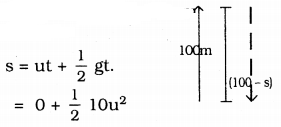(u = 0 because stone is dropped ie it starts from rest)
s = 5t2 …………. (i)
For the stone projected upward
s = ut – $$\frac{1}{2}$$ gt2
(Due to upward motion negative sign is taken)The stones will meet after; 4s
s = 5t2 = 5 × (4)2 = 80 m
The stones will be at a distance of 80 m from the top of tower or 20 mt (100 m – 80 m) from the base of the tower 20m (100m – 80m).

Question 18.
A ball thrown up vertically returns to the thrower after 6s. Find
a) the velocity with which it was thrown up
b) the maximum height it reaches, and
c) its position after 4s.
Total time taken = 6 s
Time taken to reach the maximum height = $$\frac{6}{2}$$ = 3s
(∵ Time of ascent = time)
a) From equation of motion v = u – gt
(Negative sign is taken due to upward motion)
0 = 4- 9.8 × 3 (∵ at maximum height v=0)
0 = u – 9.8 × 3
0 = u – 9.8 × 3
u = 29.4 ms-1.
(∵ at maximum height v = 0)
u = 29.4

b) From equation of motion
v2 = u2 – 2gh
0 = (29.4)2 – 2 × 9.8 × h
∴ h = $$\frac{(29.4)^{2}}{2 \times 9.8}$$ = 44.1 m
Maximum height attained by the ball is 44.1 m

c) In initial 35. the ball will rise then in next 35 it falls towards the earth
∴ The position after 4s(3 + 1)
From the equation of motion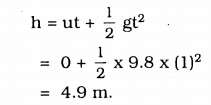∴ ie the ball will be at 4.9m from the top of the tower.

Question 19.
In what direction does the buoyant force on an object immersed in a liquid act?
The buoyant force on an object immersed in a liquid always act in upward direction.

Question 20.
Why does a block of plastic released under water come up to the surface of water.
The buoyant force on an object immersed in a liquid always act in upward direction.

Question 21.
The volume of 50g of a substance is 20 cm3. If the density of water is 1gcm-3 will the substance float or sink?
Mass of a substance = 50g
Volume of substance = 20 cm3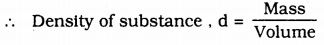= $$\frac{50}{20}$$ = 2.5 g cm-3
ie the density of the substance is greater than the density of water. So it will sink in water.

Question 22.
The volume of 500g sealed packet is 350 cm-3, will the packet float or sink in water if the density of water is 1gmcm-3? What will be the mass of the water displaced by this packet?
Mass of packet = 500g
Volume of packet = 350 cm-3∴ ie the density of packet is greater than density of water, so it will sink in water.
Mass of water displaced by the packet = Volume of packet × Density of water = 350 × 1 = 350 gm.

### KSEEB Solutions for Class 9 Science Chapter 10 Additional Questions and Answer

Fill in the blanks with suitable words.
Question 1.
The force that causes acceleration and keeps the body moving along the circular path acting towards the centre is called the _________
Centripetal force.

Question 2.
Force is the product of and _________
Mass, acceleration.

Question 3.
The ________ of an object is the force with which it is attracted towards the earth.
weight.

Question 4.
The force acting on an object perpendicular to the surface is called the ___________
thrust.

Question 5.
The upward force exerted by the water on objects is known as ________ force.
buoyant.

Question 6.
The objects of density greater than that of a liquid _________ in the liquid.
sink.

Question 7.
The value of ‘g’ in the poles is _________ than the equator.
greater.

Question 1.
Why is Newton’s law of Gravitation called universal law?
It is applicable to all bodies either they ‘ are small or big. Whether they are celestial or terrestrial.

Question 2.
Mention two factors on which the buoyant force acting on an object depends?